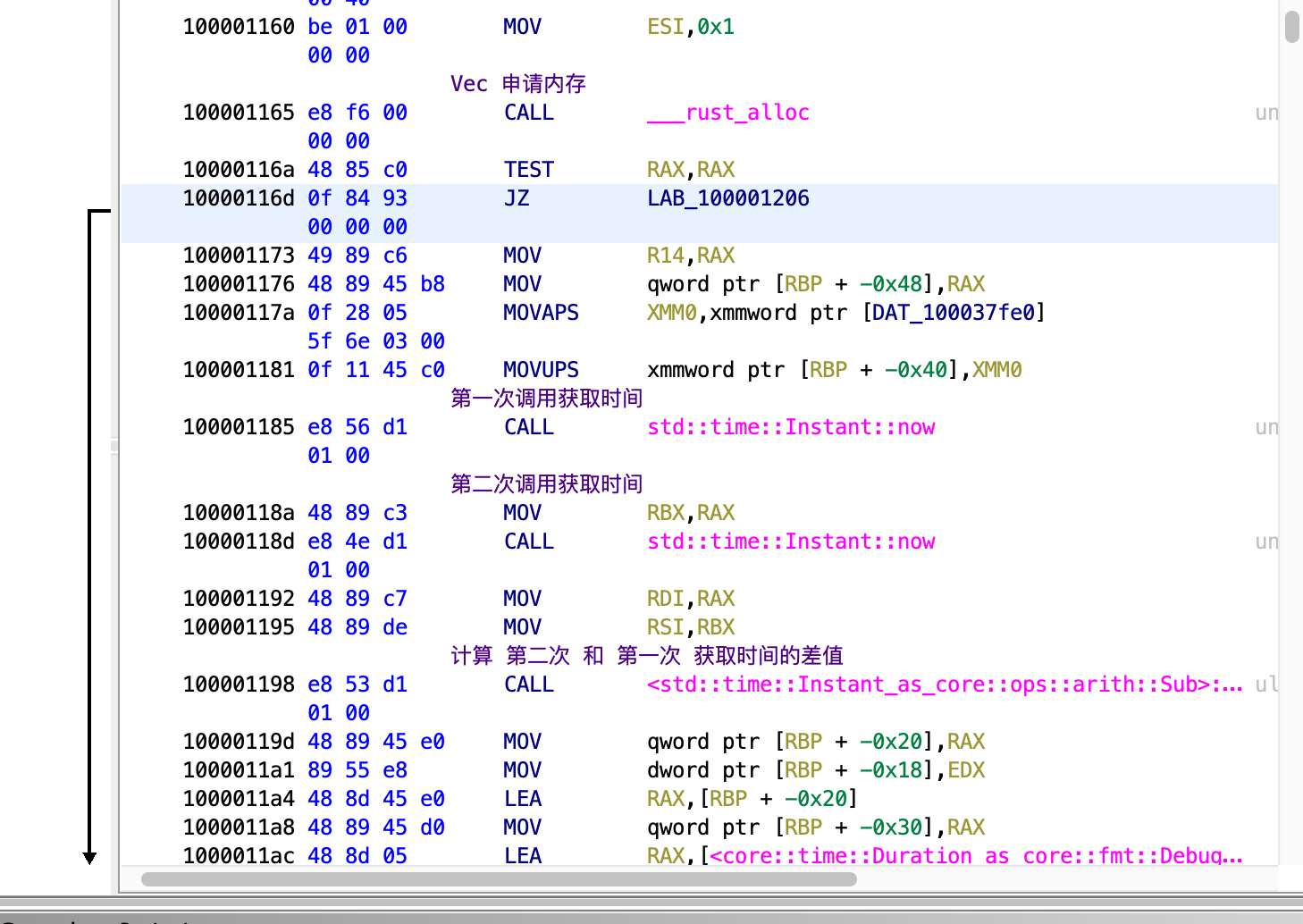# 基准测试很难¶

• CPU 性能

• 内存 读写速度

• 磁盘 I/O速度

• 网络 性能

## 内存读取测试¶

• 顺序读写(测试)

• 随机读写(测试)

let mem_size = 1024 * 1024 * 1024; // 1GB 大小的内存
let mut mem = Vec::<u8>::with_capacity(mem_size); // 分配内存到 mem 中
let ptr = mem.as_mut_ptr(); // 获取内存的基指针

// 开始时间
let start_time = time::Instant::now();
// 顺序读取 1GB 的内存
for i in 0..mem_size {
let _ = unsafe { ptr.add(i).read() };
}
// 计算并且打印出消耗的时间
let use_time = time::Instant::now() - start_time;
println!("use time: {:?}", use_time);


use time: 29ns


for i in 0..mem_size {
let _ = unsafe { ptr.add(i).read() };
}let mem_size = 1024 * 1024 * 1024; // 1GB 大小的内存
let mut mem = Vec::<u8>::with_capacity(mem_size); // 分配内存到 mem 中
let ptr = mem.as_mut_ptr(); // 获取内存的基指针
// panic if ptr is null

// 开始时间
let start_time = time::Instant::now();
// 顺序读取 1GB 的内存
// opt out: for i in 0..mem_size {
// opt out:    let _ = unsafe { ptr.add(i).read() };
// opt out: }
// 计算并且打印出消耗的时间
let use_time = time::Instant::now() - start_time;
println!("use time: {:?}", use_time);


let mem_size = 1024 * 1024 * 1024; // 1GB 大小的内存
let mut mem = Vec::<u8>::with_capacity(mem_size); // 分配内存到 mem 中
let ptr = mem.as_mut_ptr(); // 获取内存的基指针

let mut r = 0;

// 开始时间
let start_time = time::Instant::now();
// 顺序读取 1GB 的内存
for i in 0..mem_size {
// 强制使用 读取的返回值, 这样 编译器 就无法优化
}
// 计算并且打印出消耗的时间
let use_time = time::Instant::now() - start_time;
// 这儿必须 打印出: r, 否则 Rust 编译器会认为 r 这个值没有使用
// 进而导致认为: unsafe { ptr.add(i).read() } 读取语句没有任何作用(被优化)
println!("use time: {:?}, r={}", use_time, r);


use time: 738.944212ms, r=0


## 磁盘读写测试¶

• 顺序 读写

• 顺序重 读写

• 随机 读写

• 跳跃 读写

• 倒序 读写

读取 512KB 的数据仅仅使用了 0.1ms 。


## 困难来自于何处?¶

系统有过多的 抽象/优化 层。


## 参考资料¶

0

Rust Lang

Updated on 15 二月 2021

Updated on 28 二月 2021